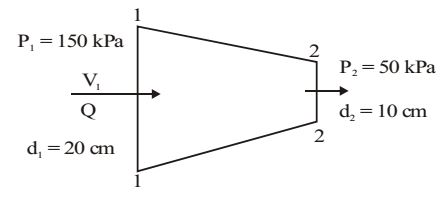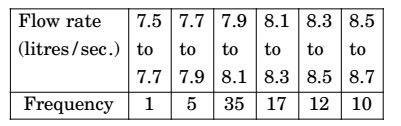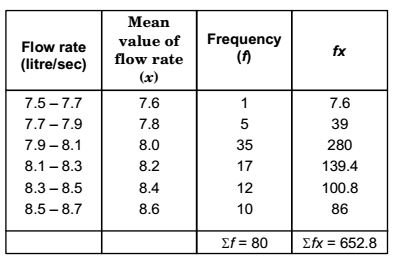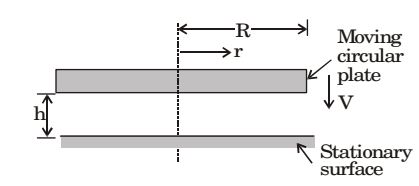## Fluid Mechanics and Hydraulic Machinery Miscellaneous

#### Fluid Mechanics and Hydraulic Machinery

1. Consider steady, incompressible and irrotational flow through a reducer in a horizontal pipe where the diameter is reduced from 20 cm to 10 cm. The pressure in the 20 cm pipe just upstream of the reducer is 150 kPa. The fluid has a vapour pressure of 50 kPa and a specific weight of 5 kN / m3 . Neglecting frictional effects, the maximum discharge (in m3 / s) that can pass through the reducer without causing cavitation is

1. Considering potential head difference = 0,
i.e z1 = z2
Apply Bernoulli’s theorem

 p + v2 = C ρg 2gp1 + v1² = p2 + v2² w1 2g w2 2g

But w1 = w2 = w = 5 (incompressible flow)
 ∴ 150 + v1² = 50 + v2² 5 2g 5 2g

 or v2² - v1² = 150 - 50 2g 5

 or v2² - v1² = 20 m ....(1) 2g

 Also, discharge, Q =π d1²v1 =π d2²v2 4 4

 or v1 =d2² =10² v2 d1 20

 or v1 = v2 ......(2) 4

From equations (1) and (2)v2² - v2²16 = 20 2g

 or 15v2² = 20 32g

or v2 = √(20 × 32g) / 15 = 20.45 m /s
 ∴ Discharge , Q =π d22v2 4

 = π (0.1)2 × 20.45 = 0.16 m3 /sec 4

##### Correct Option: B

Considering potential head difference = 0,
i.e z1 = z2
Apply Bernoulli’s theorem

 p + v2 = C ρg 2gp1 + v1² = p2 + v2² w1 2g w2 2g

But w1 = w2 = w = 5 (incompressible flow)
 ∴ 150 + v1² = 50 + v2² 5 2g 5 2g

 or v2² - v1² = 150 - 50 2g 5

 or v2² - v1² = 20 m ....(1) 2g

 Also, discharge, Q =π d1²v1 =π d2²v2 4 4

 or v1 =d2² =10² v2 d1 20

 or v1 = v2 ......(2) 4

From equations (1) and (2)v2² - v2²16 = 20 2g

 or 15v2² = 20 32g

or v2 = √(20 × 32g) / 15 = 20.45 m /s
 ∴ Discharge , Q =π d22v2 4

 = π (0.1)2 × 20.45 = 0.16 m3 /sec 4

1. The following data about the flow of liquid was observed in a continuous Chemical process plant:Mean flow rate of the liquid is

1.∴ Mean flow rate = ∑fx = 652.8 = 8.16 ∑f 80

##### Correct Option: C∴ Mean flow rate = ∑fx = 652.8 = 8.16 ∑f 80

1. Navier Stoke's equation represents the conservation of

1. Momentum

##### Correct Option: D

Momentum

1. Bernoulli's equation can be applied between any two points on a stream line for a rotational flow field. State:

1. TRUE

##### Correct Option: A

TRUE

Direction: The gap between a moving circular plate and a stationary surface is being continuously reduced, as the circular plate comes down at a uniform speed V towards the stationary bottom surface, as shown in the figure. In the process, the fluid contained between the two plates flows out radially. The fluid is assumed to be incompressible and inviscid.1. The radial component of the fluid acceleration at r = R is

 ar = Vr × ∂Vr + ∂Vr ∂r ∂t

 ar = V.r × ∂V.r+ ∂V.r2h ∂r 2h ∂t 2h

 -∂h = V ∂t

 ∴ ar = V.r × V.r + V.r ×-1 ∂h2h 2h 2 h2 ∂t

 ∴ ar = V2r + 2V2r 4h2 4h2

 ar = 3V2r 4h2

##### Correct Option: A

 ar = V.r × ∂V.r+ ∂V.r2h ∂r 2h ∂t 2h
 ∴ ar = V.r × V.r + V.r ×-1 ∂h2h 2h 2 h2 ∂t